# Coding, alphabetical

(diff) ← Older revision | Latest revision (diff) | Newer revision → (diff)

non-uniform coding

The representation of information in standard form, under which coded combinations of symbols from a given alphabet are sequentially associated with elementary syntactical units of the language of the messages (letters of the alphabet of the language). (Here, by information one means a linear string of letters.) An example of an alphabetical coding is the celebrated Morse code in which words are encoded letter by letter, and words in the alphabet of three symbols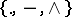(wheredenotes a blank) are associated with the letters. An example of a naturally arising coding which does not fit into the model of alphabetical coding is the decimal system of calculation. The sources of ideas for the development of alphabetical coding are the cybernetic point of view on information processes and systems on the one hand, and on the other hand the requirements of communications engineering (where the need frequently arises to transform information into a form that is more convenient in some regard); the starting point was the fundamental work of C. Shannon  published in 1948. The theory of alphabetical coding is a branch of applied mathematics that consists in the study of various mathematical models of communication channels using a variety of mathematical methods. The best studied are uniform or block codes, in which the coded combinations have fixed length. There is a well-developed mathematical apparatus for this class (see Error-correcting code). The most general abstract scheme is automatic coding, in which the correspondence is realized by a finite automaton. In practical terms, uniform coding gives, as a rule, satisfactory results; therefore, when using other coding methods these have to possess considerable merit (for example, the existence of compression of information) and their deficiencies (complications in encoding and decoding) must not be too serious.

Fundamental results of a general character can be stated only for the case of letter by letter alphabetical coding (when the coding automaton has only one internal state), since the known generalizations have no fundamental significance, while investigations in connection with other models have not yet attained any significant theoretical development. The following model of a communication channel is considered:Figure: c022880a

Source of informationcoding schemedecoding schemereceiver

Here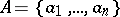is the alphabet of the communication channel, that is, the list of signals that can be transmitted along the channel,is the duration of the signal, and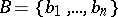is the alphabet of the language of the messages. In the simplest case, the source of information is a probabilistic scheme at the output of which one of the letters ofappears at each moment of discrete time, the probabilities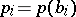of appearance of the letters being independent of time. A coding schememaps the letters ofinto words of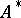(whereis the monoid generated by),, and the words of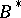are encoded letter by letter:. Thus,is completely determined by the code. Ifis a one-to-one mapping, then the problem of the decoding scheme is to recover the transmitted message by realizing the mapping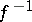.

The rate of information transmission (cf. Information, transmission rate of) and the complexity of decoding determined by the choice of the codeare factors to be estimated for the model. The transmission rate is characterized quantitatively by the mathematical expectation of the time required for the transmission of a single letter of the message: The duration of the transmission of a letter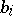iswhere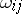is the number of occurrences of the letter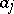in the word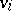, and the required mathematical expectation is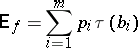(is also called the cost of the coding). In the general case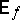depends on the structure of the code, which is described by a structure polynomial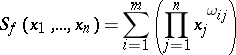— the generating function, which enumerates the words of the code from their composition. In the particular case, one has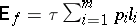, i.e.is determined only by the spectrum of the lengths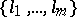of the words of the code, where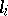is the length of the word. In this case, the problem of the selection of an optimal code (that is, a cost-minimizing one) has been completely solved; it has been proved  that a necessary and sufficient spectral condition for the existence of a unique decodable code is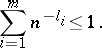(1)

It has been shown in  that, together with (1), the conditionis a necessary and sufficient condition for the existence of a code with a given spectrum having the so-called property of self-synchronization, meaning that an error in decoding is automatically located with probability 1.

The following qualitative measure of the complexity of decoding is of greatest interest from an abstract point of view: The delay in the decoding should have the property of finiteness, meaning that it is possible to effect the decoding by a finite automaton. (Quantitatively estimating the complexity of a circuit realization by automata goes beyond the scope of coding theory.) The so-called prefix codes (that is, codes for which no word ofis the initial segment of another word in) all have this property. It has been proved  that any code for whichis one-to-one is spectrally equivalent to some prefix code. One has a relatively good overview of prefix codes, and the successful results obtained for the case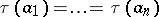are explained by this.

In the general case, algorithmic solutions are known for the problem of recognizing the one-to-one property of a coding and the property of finiteness of delay of the decoding. The one-to-one property ofcan be regarded as a minimal level of noise stability of the code. There are algorithmic solutions for the problem of calculating the actual correcting ability of an arbitrary code in the general case as well as under the supplementary requirement that the decoding delay be finite. The class of free (that is, uniquely decodable) codes has a highly complicated structure, but extremal requirements on the codes often lead to substantial restrictions. For example, it has been shown that for codes that are maximal with respect to inclusion (for which inequality (1) becomes an equality; these are called complete because only for them the alphabet of the channel is used fully in the sense that any word ofis part of some encoded message) the finite delay property holds if and only if the code is prefix.

How to Cite This Entry:
Coding, alphabetical. Encyclopedia of Mathematics. URL: http://encyclopediaofmath.org/index.php?title=Coding,_alphabetical&oldid=18635
This article was adapted from an original article by Al.A. Markov (originator), which appeared in Encyclopedia of Mathematics - ISBN 1402006098. See original article